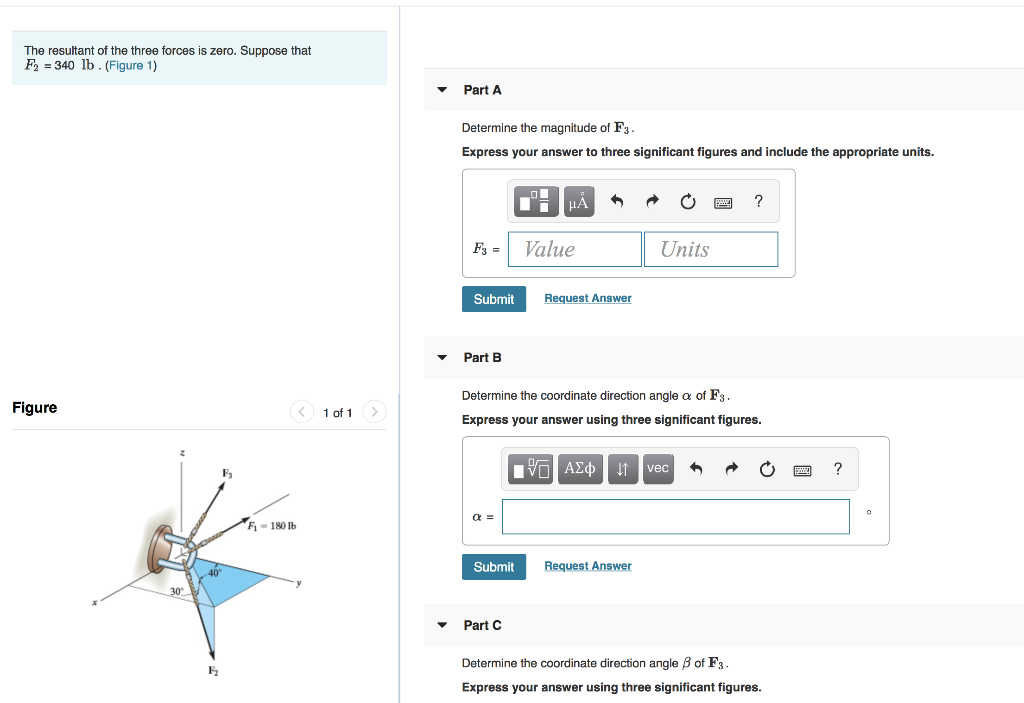Home / Answered Questions / Other / the-resultant-of-the-three-forces-is-zero-suppose-that-f2-340-lb-figure-1-part-a-determine-the-magni-aw965

# (Solved): The Resultant Of The Three Forces Is Zero. Suppose That F2 = 340 Lb. (Figure 1) Part A Determine The...The resultant of the three forces is zero. Suppose that F2 = 340 lb. (Figure 1) Part A Determine the magnitude of F3. Express your answer to three significant figures and include the appropriate units. HÃ…R O E? Value Units Fs = Submit Request Answer Part B Figure < 1 of 1 > Determine the coordinate direction angle a of F3. Express your answer using three significant figures. o A vec R O O ? F-180 lb Submit Request Answer . 30 Part Determine the coordinate direction angle B of F3. Express your answer using three significant figures.

We have an Answer from Expert